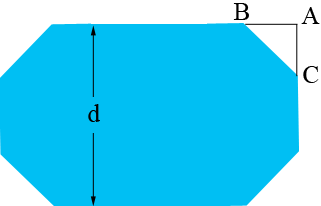SEARCH HOMEMath Central Quandaries & QueriesQuestion from Roberto: I am trying to build a "ring" for our remote control boats, using irrigation pipe. I'd like to use full 20' long sticks for the long sides and use eight of what we call 45s, which are actually 135' fittings to make the six short sides. If I know the length of the two long sides, what would be the formula to find the exact lengths of the short sides? Thank you.Hi Roberto,

What I see is thiswhere each of the long sides is 20 feet long and the measure of each of the internal angles is 135 degrees.If this is correct then I need more information to determine the lengths of the six shorter sides. In particular do you know the distance between the long sides?

Penny

Roberto wrote back.

I was hoping to divide two twenty foot sticks into three equal lengths of 6.67', using the 135 degree fittings, so I honestly don't know what the distance would be between long sides.

Sides of length 6.67' will certainly work, I just thought that the width of the "ring" might be important. Once you know one you can determine the other.

Suppose $d$ is the distance between the long sides as in the diagram below and $x$ is the lengths of the short sides.The triangle $ABC$ is an isosceles right triangle with the length of the hypotenuse $x$ feet and thus Pythagoras Theorem says that the length of $AC$ is $\frac{x}{\sqrt 2}$ feet. Thus

$d = 2 \times |AC| + x = \sqrt 2 x + x = \left(1 + \sqrt 2 \right) x.$

Hence if $x = 6.67$ feet then $d = 16.10$ feet.

Penny* Registered trade mark of Imperial Oil Limited. Used under license.Math Central is supported by the University of Regina and the Imperial Oil Foundation.